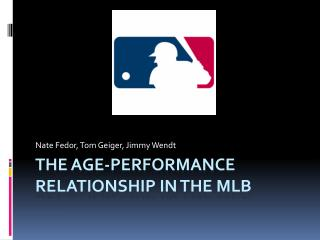Download PresentationThe age-performance relationship in the mlbThe age-performance relationship in the mlb - PowerPoint PPT Presentation

Download PresentationThe age-performance relationship in the mlb
An Image/Link below is provided (as is) to download presentation

Download Policy: Content on the Website is provided to you AS IS for your information and personal use and may not be sold / licensed / shared on other websites without getting consent from its author. While downloading, if for some reason you are not able to download a presentation, the publisher may have deleted the file from their server.

- - - - - - - - - - - - - - - - - - - - - - - - - - - E N D - - - - - - - - - - - - - - - - - - - - - - - - - - -
Presentation Transcript

1. Nate Fedor, Tom Geiger, Jimmy Wendt The age-performance relationship in the mlb

2. “Measuring the Experience-Productivity Relationship: The Case of Major League Baseball” by Gregory Krohn (1983) • Krohn’s research question • Avgi(t) = β0 + β1Agei(t) + β2[Agei(t)2] + µi(t) where i = 1, 2, …, N; t = 1, 2, …, T; Avgi(t) = the batting average of player i in year t; Agei(t) = the age of player i in year t; µi(t) = error term for observation i in year t • Krohn found the age of peak performance to be 28 years of age

3. Hypothesis • The age of peak performance has increased since 1983 due to: • Technology • Training techniques • Steroids

4. The Data • 10 “Steroid Era” sluggers • 10 “Historical” sluggers • Season by season stats (minimum of 200 at-bats)

5. The Players “Historical” “Steroid Era” • Mickey Mantle • Frank Robinson • Eddie Mathews • Willie Mays • Ernie Banks • Harmon Killebrew • Hank Aaron • Carl Yastrzemski • Stan Musial • Willie Stargell • Barry Bonds • Mark McGwire • Sammy Sosa • Rafael Palmeiro • Juan Gonzalez • Jose Canseco • Albert Belle • Greg Vaughn • Ken Caminiti • Jeff Bagwell

6. Models • Avgi(t) = β0 + β1Agei(t) + β2[Agei(t)2] + µi(t) • AB/HRi(t) = β0 + β1Agei(t) + β2[Agei(t)2] + µi(t)

7. “Historical” Player Results The regression equation is AVG = 175 + 9.81 Age - 0.194 Age^2 Predictor Coef SE Coef T P Constant 175.31 53.87 3.25 0.001 Age 9.809 3.607 2.72 0.007 Age^2 -0.19370 0.05853 -3.31 0.001 S = 33.6690 R-Sq = 17.2% R-Sq(adj) = 16.4% By taking the first derivative of the regression equation and then setting it equal to zero, we can calculate the peak age Peak age (for batting avg) = 25 years

8. “Historical” Results (Cont.) The regression equation is AB/HR = 105 - 5.80 Age + 0.0961 Age^2 Predictor Coef SE CoefT P Constant 104.66 14.54 7.20 0.000 Age -5.8010 0.9629 - 6.02 0.000 Age^2 0.09612 0.01551 6.20 0.000 S = 7.84034 R-Sq = 17.7% R-Sq(adj) = 16.8% Peak age (for AB/HR) = 30 years

9. “Steroid Era” Results The regression equation is AVG = 39.2 + 15.5 Age - 0.245 Age^2 Predictor Coef SE Coef T P Constant 39.21 98.89 0.40 0.692 Age 15.525 6.527 2.38 0.019 Age^2 -0.2446 0.1058 -2.31 0.022 S = 34.2338 R-Sq = 4.0% R-Sq(adj) = 2.7% Peak age (for batting avg) = 32 years

10. Class Activity • P:\temp\0 Nate Tom Jimmy\class activity.MTW • We will estimate the peak age for AB/HR for “Steroid Era” players

11. Conclusion • Peak age of performance did increase • Training? • Technology? • Steroids? • Coaching? • R-Sq values are very low • Omitted variable bias • Talent, injuries, coaching. Ideas? • More explanatory variables needed • Larger random sample data set.

12. HW Problem In class we estimated the regression equation AB/HR = 104 - 4.72 Age + 0.0618 Age^2 for modern day sluggers. Barry Bonds was born on July 24 1964. If he were playing this season, estimate what his at-bats per home run ratio would be.